# A zest of number theory

I just encountered an amazing number theoretic result. It is probably very well known, but for those who never saw it it’s quite something, so I thought I would share it.

Let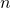be a positive integer. A partition ofis a multiset of positive integers such that the sum of its elements is equal to(for example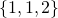is a partition of). The partition functionis defined as the number of partitions of. The amazing result that I want to tell you about is the formula for the asymptotic equivalent of. Before giving away the formula let’s try to get some intuition about the order of magnitude one can expect. For this let me first look at the simpler quantity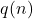, defined as the number of multiset of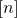withelements (for example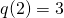). With the stars and bars argument (thanks to Luc Devroye for reminding me about this elementary combinatorial technique!) one can easily see that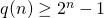. Moreover it is also easy to see that there is an injection from multisets ofwith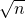elements to partitions of(observe that the sum of the elements of such a multiset is necessarily smaller than). ThusA more involved argument, based on Young Diagram representation of partitions, shows that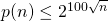(don’t quote me on the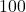, I think it’s correct but I have only rough calculations here).

Now here we go for the asymptotic formula, discovered by Hardy and Ramanujan in 1918:In my opinion this is a beautiful connection between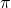,, and a basic property of integers. The proof is based on the circle method, an elementary technique in complex analysis (see here for the details).

This entry was posted in Theoretical Computer Science. Bookmark the permalink.